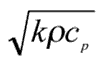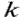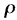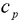The Science

C-Therm’s technology is based on the modified transient plane source technique. It employs a one-sided, interfacial heat reflectance device that applies a constant current heat source to the sample. The interfacial sensor heats the sample by approximately 1-3°C during the testing. The sample absorbs some of the heat, and the rest causes a temperature rise at the sensor interface.

The rate of the increase in temperature at the sensor surface is inversely proportional to the ability of the sample to transfer heat. Thermal conductivity and effusivity are measured directly, providing a detailed overview of the thermal nature of the sample material.

Thermal Conductivity

Thermal conductivity is a physical property of a material that characterizes the ability of that substance to transfer heat. The value of thermal conductivity determines the quantity of heat passing per unit of time per unit area at a temperature drop of 1-degree C per unit length. In the limit of infinitesimal thickness and difference in temperature, the fundamental law of heat conduction is:

Where:

is a measure of the heat flow

A  is the cross sectional area

dT/dX is the temperature / thickness gradient

is defined as the thermal conductivity\

Thermal conductivity differs with each substance and may depend on the structure, density, humidity, pressure and temperature. Materials having a large thermal conductivity value are good conductors of heat; one with a small thermal conductivity value is a poor heat conductor i.e. good insulator. Hence, knowledge of the thermal conductivity value (units W/m•K) allows for quantitative comparisons to be made between the thermal insulation efficiencies of different materials.

Learn the Thermal Conductivity of various substances.

Effusivity

Effusivity combines thermal conductivity, density and heat capacity – is a heat transfer property present in all materials in all formats – solid, liquid, paste, powder and gas.  Effusivity is the property that dictates the interfacial temperature when two semi-infinite objects at different temperature touch. Effusivity combines thermal conductivity, density and heat capacity into one value.

Effusivity =Where:= Thermal Conductivity (W/m•K)= Density (kg/m3)= Heat Capacity (l/kg•K)